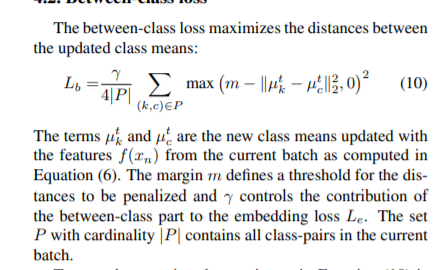# If the calculation step of the loss function is independent of the model output, then is the back propagation will update the model parameters?

This particular loss function is derived from this paper in sections 4.1 and 4.2:

paper

In this paper, two functions are proposed to process fine-grained image classification：

The first loss functions is basically the same as center_loss:

This is the definition center_loss, where f(xn) is the output of the network

But the problem is the second loss function that it definesIt can be seen that the calculation of the loss function is only related to the center in center_loss, and the subset of the category is selected through target. Both labels in the subset are different. This loss function is used to highlight differences between classes

Because centerloss update center is after the loss.backward(), so the second loss function is same as that. So the output of the model only participates in the center update of the second loss function after loss.backward(),this should not affect the model’s parameters update (I guess), so the second loss function should not have an effect on model parameters updating .

But the gradient of the function to the output of the model is still mentioned in the paper：

According to my knowledge of the chain rule, this should have no gradient to the output of the model.

Can someone help me explain how this gradient is calculated？

Thanks！